# 15个常用excel函数公式_（干货）EXCEL常用函数公式大全及举例

(一)函数语法

(二)运算符

1. 公式运算符：加(+)、减(-)、乘(*)、除(/)、百分号(%)、乘幂(＾)

2. 比较运算符：大与(>)、小于(=) 、不等于(<>)

3. 引用运算符：区域运算符(：)、联合运算符(,)

(三)单元格的相对引用与绝对引用

$A1 锁定第A列 A$1 锁定第1行

$A$1 锁定第A列与第1行

(一)数学函数

1. 求和 =SUM(数值1,数值2,……)

2. 条件求和 =SUMIF(查找的范围,条件(即对象),要求和的范围)

(2)=SUMIF(A1:A4,”<300”,C1:C4)

3. 求个数 =COUNT(数值1,数值2,……)

(2) =COUNT(A1:C4) 函数意思：第A1栏至C4栏求个数

4. 条件求个数 =COUNTIF(范围,条件)

(2) =COUNTIF(A1:C4,”>=1000”)

5. 求算术平均数 =AVERAGE(数值1,数值2,……)

(2) =AVERAGE(A1:A4)

6. 四舍五入函数 =ROUND(数值,保留的小数位数)

7. 排位函数 =RANK(数值,范围,序别) 1-升序 0-降序

(2) =RANK(A1,A1:A4,0)

8. 乘积函数 =PRODUCT(数值1,数值2,……)

9. 取绝对值 =ABS(数字)

10. 取整 =INT(数字)

(二)逻辑函数

=IF(A1>A2,1,0)=1(真)

=IF(A1

=IF(A1>A2,IF(A3>A4,8,9),1)=9

(三)文本函数

1. 截取函数： =LEFT(文本,截取长度)—从左边截取

=RIGHT(文本,截取长度)—从右边截取

=MID(文本,开始位,截取长度)—从中间截取

2. 计算字符长度：LEN(文本)—文本的长度

3. 合并字符函数：CONCATENATE(文本1,文本2,…)

4. 在字符串中查找特定字符：FIND(文本,范围,数值)—数值表示查找第几个

5. 比较两个字符是否完全相符：EXACT(文本1,文本2)

=EXACT(“a”,“a”)=TRUE

=EXACT(“a”,“ab”)=FALSE

=EXACT(“a”,“A”)=FALSE

6. 将数值转化为文本：TEXT(数值,参数) )—参数一般为0

7. 将数值型字符转换为数值：VALUE(数值文本)

(四)判断公式

1. 把公式产生的错误显示为空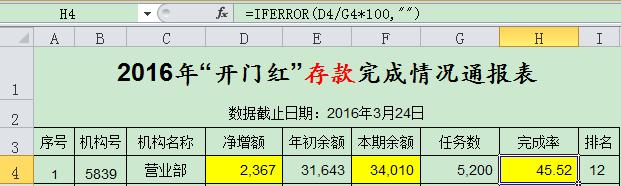2. IF多条件判断返回值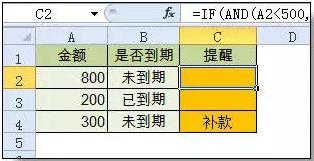(五)统计公式

1. 统计两个表格重复的内容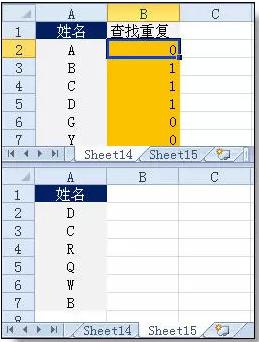2. 统计不重复的总人数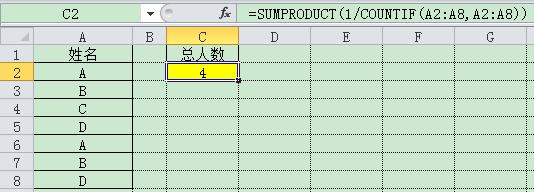(六)求和公式

1. 隔列求和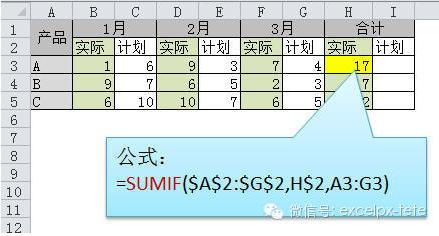2. 单条件求和

3. 单条件模糊求和

4. 多条件模糊求和

5. 多表相同位置求和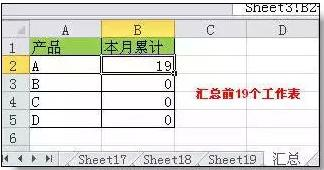6. 按日期和产品求和

(七)查找与引用公式

1. 单条件查找公式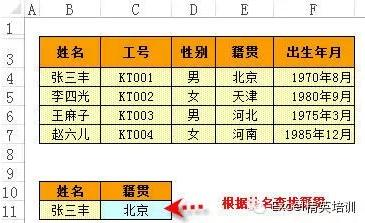2. 双向查找公式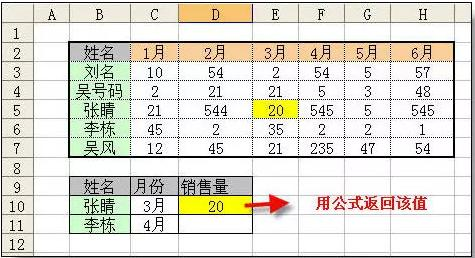3. 查找最后一条符合条件的记录。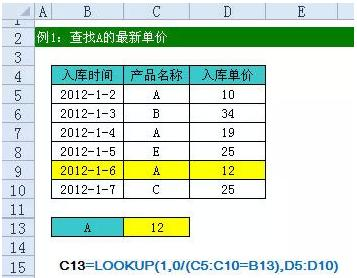4. 多条件查找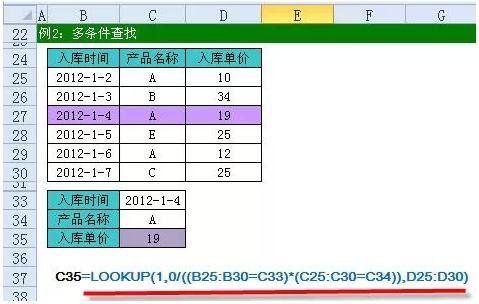5. 指定区域最后一个非空值查找

6. 按数字区域间取对应的值

10-26
11-0508-09
07-04
10-2210
11-11207
11-21132
04-18215
11-12
01-041万+
08-19230
11-21457
12-2638
11-2115
05-08276
11-2275点击重新获取扫码支付余额充值